# Identifier detection in datasets

## By Wajdi FARHANI

LumenAI is developing an innovative and interactive solution for real time unsupervised learning that allows data scientists to understand the structure of their data with the minimum of prior expertise knowledge.

Users will be able to upload their datasets in .csv format and LumenAI's clustering algorithms will try to find the optimal number of clusters and their positions. A summary of each cluster will be returned at the end of the task together with an interactive visualization that the data scientist can use to perfectly understand his dataset.

For UX reasons, we need to reduce the number of required actions from the user before launching the clustering task. To do so, an automatic preprocess of the uploaded dataset starts at the end of the upload. Operations like handling missing data and transforming non-numeric columns are performed in this first step of the clustering pipeline.

IDs detection

The preprocessing step remains incomplete as it does not deal with the case of senseless numeric columns like the famous nominal (digital) identifiers. This columns should be removed from the dataset as they can seriously impact the relevance of the clustering results!

So we need a model that can detect nominal Identifiers so we can eliminate them after the confirmation of the user.

Caution: even if the confirmation of excluding identifiers from the clustering task still a required action, the automatic detection of IDs will save the user time as he manually check/uncheck is required.

What's an ID ?

An identifier is a name or a number that identifies a person, a category or an object. Datasets have often at least one column that identifies the objects concerned by the each row (attributes).

An identifier should be unique for each object. So why is it a problem to detect identifiers since a simple check of the distinct values taken by a variable can determine if it's an identifier?

Good question ! Unfortunately the problem is harder in our case since this rule is not always respected as we can find several lines that concern the same object in many datasets.

For example, in the case of data related to visitors behavior on an e-commerce website, several actions can be assigned to the same visitor, which will be reflected by an identifier value that is repeated several times throughout the dataset.
A machine learning based model can be the solution if we consider the problem as a classification task (1: identifier and 0: non-identifier attribute).

Feature engineering

To find features to use we tried to find what are the specificities of an identifier in a datasets. In other words, how an identifier may be different from any other attribute of the dataset.

• Distinct values:​ Even if the identifier is not always unique as already mentioned, the ratio of distinct values in a column can give an idea of its "identifier character".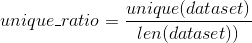• Entropy: Entropy in general "characterizes the degree of disorganization, or unpredictability of the information content of a system" (wiki). The identifier is often a variable with a large entropy since its values are often assigned in a random and uniform way to the objects it designates. This information can therefore be useful for classifying attributes.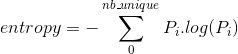• Correlation with other variables: Correlation gives an idea about the degree of dependence between two variables. It is therefore reasonable to think that the correlation between one column and the others can give an idea that it is an identifier.
We will use two methods to calculate the correlation:
• Pearson correlation: It's the "standard" correlation defined as the ratio between the covariance of two    variables and the product of their standard deviations.
• Spearman correlation:  regarding the mechanisms for generating IDs and QIDs, one can think that  the correlation between identifiers and other variables may be stronger when it is calculated not between the values taken by the nominal identifiers and those of the other variables but rather by ranks of its values. Hence the need for the so-called Spearman correlation.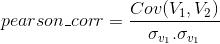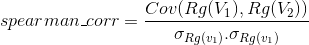• Position of the attribute in the dataset: For simplicity's sake and without it being a convention, it is often preferable that the columns that serve as identifiers are placed at the beginning of the list of columns.
• Variance
• Minimum
• Maximum
• Mean
• Median
• 25th and 75th percentile

Data collection

The proposals of attributes described above are based on assumptions that must be verified using real data. But how can we find a dataset that contains informations about other datasets ?

After a long and unsuccessful search in the open access databases, we decided to create our own columns dataset by following the following steps:

• Manually collect datasets with identifiers that can be easily detected from their names.
• Divide each dataset into 10 sub-datasets in order to increase the number of observations
• Compute the above variables from each sub-dataset (corr, min, max, entropy etc.)
• Add a label column to the dataset

To create a train and test datasets, we need to compute the above variables for each column of each sub-dataset.

Code to compute that variables is available in this

Caution : To avoid any confusion, it is important to clarify that our objective here is to build a dataset where each row corresponds to a column in another dataset.

Identifier detection

Preprocessing

The dataset we obtained contains 2000 lignes and 14 columns thanks to the data augmentation trick we performed on collected datasets by dividing each one into 10 sub-datasets.

The first problem we faced was the unbalanced labels which was expected as datasets contains often few identifiers among their columns.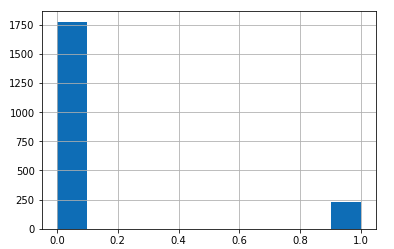This code can reduce the unbalance:

After reducing the number of non-id rows: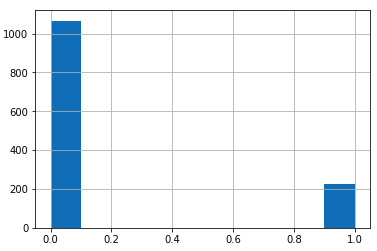In order to validate (or not) the assumptions made above, let's look at the correlations between the variables and the label: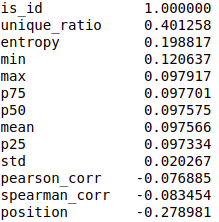Correlations validate many of our assumptions, particularly about the importance of to the number of unique values, entropy, position and minimum values of identifiers.
From the list of variables we will choose the 5 most correlated with our target (is_id).

Algorithm choice and results

Regression or classification ?

Even if it is a classification problem, we prefer consider it as a regression problem since our objective is to associate a score to each column of the dataset that reflects the probability that it is an identifier. If the model associates a high score to a column, we will ask the user to confirm this "intuition".

The probability threshold from which we will consider that the model have detected an identifier is a parameter to be set according to the desired sensitivity.

Objective

Missing an identifier means that a senseless attribute can be used in the clustering task which will seriously impact the algorithm's output. To avoid this risk, false negatives have to be minimized.

Minimizing false negatives means maximizing the recall (rate of detected true labels from to the total number of true labels in the dataset) but it is important to watch the model's accuracy too (rate of detected true labels relative to the number of positive predictions of the model) so we can avoid too many confirmation requests to the user.

Test and validation

Given the small size of our dataset (2k lines), the traditional split TRAIN:0.8 - TEST:0.2 may lead to an overfitted model. Cross-validation should be used to avoid overfitting.

Cross-validation consists in dividing the learning set into k sub-sets (folds) and, at each iteration, the model is trained using the k-1 sub-sets and validation is done using the remaining sub-sets. The final score of the model will be the average of the scores of each iteration.

In order to use cross-validation of the scikit-learn package, we need to override the prediction method of the regression algorithms we are using to make them return binary result as the validation phase is waiting to have binary labels like in the test set.

Results

The table below present the results obtained for the 4 regression-algorithms used so far: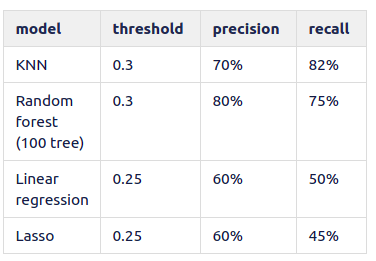All Posts
×

Almost done…

We just sent you an email. Please click the link in the email to confirm your subscription!